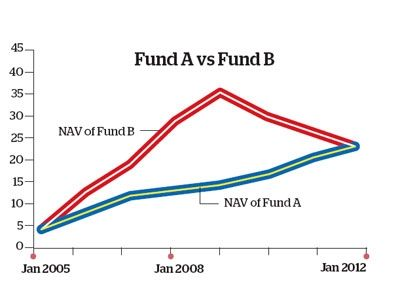# Articles

 Tweet
Articles

Mutual Funds - How to calculate returns from a mutual fund ?
12-Apr-2012

### diclofenac

diclofenac sebtiz13.fr
an, times, serif">How can an investor evaluate the performance of a fund?One of the simplest ways is to look at the historical analysis of returns. For this, one can either consider point-to-point returns or rolling returns. Let�s look at what these terms mean, and which one is a better measuring tool. Point to point returns: These returns are calculated by considering the NAVs at two points in time-entry date and exit date. Suppose you invested in the growth option of a mutual fund scheme in January 2005 at a NAV of 12. Now, if the NAV were to rise to 32 on the exit day, say, January 2012, using point-to-point returns you�ll find that your fund has generated an absolute return of 166.67%.

To know how your investment has grown on an annual basis, you�ll need to check the compounded annual growth rate (CAGR), in this case, 15.04%. Though CAGR can be calculated for any time period, a simple point-to-point return is preferred when the holding period is less than one year and CAGR is ideal for longer holding periods. Though it�s easy to calculate the point-to-point return and is extensively used to analyse fund performance, be warned that it�s not a fool-proof method. It fails to determine the consistency of the historical returns. If you consider the graph, both Fund A and Fund B have the same entry and exit NAVs. So a simple CAGR calculation would yield identical results, that is, 10.41%, while the absolute return for both funds is 100%. What�s different between the two funds is the consistency of their respective performance-Fund A is consistently rising whereas Fund B, after showing a robust growth for the first four years, declines consistently after 2009.

Clearly, Fund A is a better choice, which you�d never know by relying on the point-to-point return measures. Rolling returns: This is where rolling returns come to the rescue. In this case, returns are calculated on a continuous basis for each defined interval, which can be days, weeks, months, quarters, even years. Consider the calculation of yearly rolling returns. Going back to the first example (mutual fund with the 2005-12 holding period), the yearly return is calculated on a daily basis, from 1 January 2005, to 1 January 2006, then 2 January 2005 to 2 January 2006, and so on, till the end of the time period, that is 1 January 2011 to 1 January 2012. Assuming 250 trading days in a year, this exercise will throw up around 1,500 yearly point-to-point returns, and the average of all these returns is used as the one-year rolling return. This figure can be compared with the category average rolling return.

So, if the fund has delivered a 12% yearly rolling return, while its category average one-year rolling return is 14%, it implies that the fund has fared worse than its average. An analysis of rolling returns also throws up other relevant statistics, the most important ones being the maximum return (highest of the 1,500 yearly returns) and the minimum return (lowest figure). The maximum/minimum return not only helps determine the consistency of a fund�s performance, but also assess its best and worst periods (years, months, quarters) in terms of returns. Rolling returns can be calculated for any interval.

 If the defined interval is three years, the point-to-point returns will be calculated from 1 January 2005 to 1 January 2008, and so on, till the end of the holding period. The average of all the figures will help arrive at the three-year rolling return. Hence, calculating rolling returns is a better way to ferret out consistent performers.Source : ET Bureau back